# Electrostatics Practice Flashcards

Set Details Share
created 5 years ago by ssdesa_100
2,095 views
updated 5 years ago by ssdesa_100
Subjects:
physics
Page to share:
Embed this setcancel
COPY
code changes based on your size selection
Size:
X

1

A solid conducting sphere is given a positive charge Q. How is the charge Q distributed in or on the sphere?
(A) It is concentrated at the center of the sphere.
(B) It is uniformly distributed throughout the sphere.
(C) Its density decreases radially outward from the center.
(D) It is uniformly distributed on the surface of the sphere only

(D)

2

A parallel–plate capacitor is charged by connection to a battery. If the battery is disconnected and the separation
between the plates is increased, what will happen to the charge on the capacitor and the voltage across it?
(A) Both remain fixed.

(B) Both increase.

(C) The charge increases and the voltage decreases.

(D) The charge remains fixed and the voltage increases.

(D)

3

One joule of work is needed to move one coulomb of charge from one point to another with no change in
velocity. Which of the following is true between the two points?
(A) The current is one ampere.
(B) The potential difference is one volt. (C) The electric field strength is one newton per coulomb.
(D) The electric field strength is one joule per electron.

(B)

4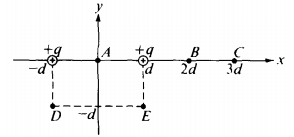Two positive charges of magnitude q are each a distance d from the origin A of a coordinate system as shown
above.

At which of the following points is the electric field least in magnitude?
(A) A (B) B (C) C (D) D

(A)

5Two positive charges of magnitude q are each a distance d from the origin A of a coordinate system as shown
above.

At which of the following points is the electric potential greatest in magnitude?
(A) A (B) B (C) C (D) D

(A)

6

A parallel–plate capacitor has a capacitance Co. A second parallel–plate capacitor has plates with twice the area
and twice the separation. The capacitance of the second capacitor is most nearly
(A) ¼Co (B) Co (C) 2Co (D) 4Co

(B)

7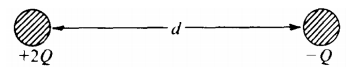Two identical conducting spheres are charged to +2Q and –Q. respectively, and are separated by a distance d (much greater than the radii of the spheres) as shown above. The magnitude of the force of attraction on the left sphere is F1. After the two spheres are made to touch and then are re-separated by distance d, the magnitude of
the force on the left sphere is F2.

Which of the following relationships is correct?
(A) 2F1 = F2 (B) F1 = F2 (C) F1 = 2F2 (D) F1 = 8 F2

(D)

8

The capacitance of a parallel–plate capacitor can be increased by increasing which of the following?
(A) The distance between the plates (B) The charge on each plate (C) The area of the plates
(D) The potential difference across the plates

(C)

9

A hollow metal sphere of radius R is positively charged. Of the following distances from the center of the sphere, which location will have the greatest electric field strength?
(A) 0 (center of the sphere) (B) 3R/2 (C) 2R
(D) None of the above because the field is of constant strength

(B)

10

Two isolated charges, + q and – 2q, are 2 centimeters apart. If F is the magnitude of the force acting on charge –2Q, what are the magnitude and direction of the force acting on charge +q?

Magnitude Direction
(A) (1/2) F Toward charge – 2q
(B) 2 F Away from charge –2q
(C) F Toward charge – 2q
(D) F Away from charge – 2q

(C)

11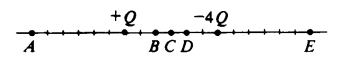Charges +Q and -4Q are situated as shown above. The net electric field is zero nearest which point?

1. A
2. C
3. D
4. E

(A)

12

A positive charge of 10–6 coulomb is placed on an insulated solid conducting sphere. Which of the following is true?
(A) The charge resides uniformly throughout the sphere.
(B) The electric field in the region surrounding the sphere increases with increasing distance from the sphere.
(C) An insulated metal object acquires a net positive charge when brought near to, but not in contact with, the
sphere.
(D) When a second conducting sphere is connected by a conducting wire to the first sphere, charge is
transferred until the electric potentials of the two spheres are equal.

(D)

13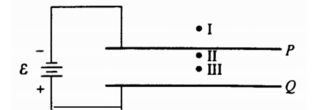Two large parallel conducting plates P and Q are connected to a battery of emf E, as shown above.
A test charge is placed successively at points I, II, and III. If edge effects are negligible, the force on the charge when it is at point III is
(A) of equal magnitude and in the same direction as the force on the charge when it is at point I
(B) of equal magnitude and in the same direction as the force on the charge when it is at point II
(C) much greater in magnitude than the force on the charge when it is at point II, but in the same direction
(D) much less in magnitude than the force on the charge when it is at point II, but in the same direction

(B)

14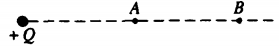The diagram above shows an isolated, positive charge Q. Point (B) is twice as far away from Q as point A. The ratio of the electric field strength at point A to the electric field strength at point B is
(A) 8 to 1

(B) 4 to 1

(C) 2 to 1

(D) 1 to 2

(B)

15

Which of the following is true about the net force on an uncharged conducting sphere in a uniform electric field?
(A) It is zero.

(B) It is in the direction of the field.

(C) It is in the direction opposite to the field.

(D) It causes the sphere to oscillate about an equilibrium position.

(A)

16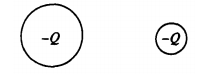Two conducting spheres of different radii, as shown above, each have charge –Q. Which of the following occurs when the two spheres are connected with a conducting wire?
(A) No charge flows.
(B) Negative charge flows from the larger sphere to the smaller sphere until the electric potential of each sphere is the same.
(C) Negative charge flows from the smaller sphere to the larger sphere until the electric field at the surface of each sphere is the same.
(D) Negative charge flows from the smaller sphere to the larger sphere until the electric potential of each sphere is the same.

(D)

17

Two parallel conducting plates are connected to a constant voltage source. The magnitude of the electric field between the plates is 2,000 N/C. If the voltage is doubled and the distance between the plates is reduced to 1/5 the original distance, the magnitude of the new electric field is
(A) 800 N/C

(B) 1,600 N/C

(C) 2,400 N/C

(D) 20,000 N/C

(D)

18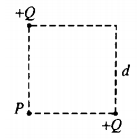The figure above shows two particles, each with a charge of +Q, that are located at the opposite corners of a square of side d. 18. What is the direction of the net electric field at point P ?
(A) ↖

(B) ↗

(C) ↙

(D) ↘

(C)

19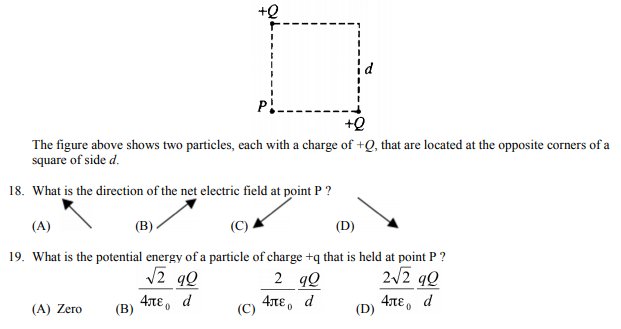The figure above shows two particles, each with a charge of +Q, that are located at the opposite corners of a square of side d.

What is the potential energy of a particle of charge +q that is held at point P ?
(A) Zero

(B)

(C)

(D)

(C)

20

Two parallel conducting plates, separated by a distance d, are connected to a battery of emf E. Which of the following is correct if the plate separation is doubled while the battery remains connected?
(A) The electric charge on the plates is doubled.
(B) The electric charge on the plates is halved.
(C) The potential difference between the plates is doubled.
(E) The capacitance is unchanged.

(B)

21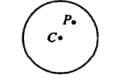The hollow metal sphere shown above is positively charged. Point C is the center of the sphere and point P is any other point within the sphere. Which of the following is true of the electric field at these points?
(A) It is zero at both points.
(B) It is zero at C, but at P it is not zero and is directed inward.
(C) It is zero at C, but at P it is not zero and is directed outward.
(D) It is not zero at either point.

(A)

22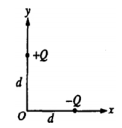Charges –Q and +Q are located on the x– and y–axes, respectively, each at a distance d from the origin O, as shown above.
What is the direction of the electric field at the origin O ?

(A) →

(B) ↖

(C) ↘

(D) ↙

(C)

23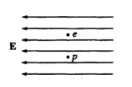An electron e and a proton p are simultaneously released from rest in a uniform electric field E, as shown above. Assume that the particles are sufficiently far apart so that the only force acting on each particle after it is released is that due to the electric field. At a later time when the particles are still in the field, the electron and
the proton will have the same
(A) direction of motion

(B) speed

(C) magnitude of acceleration

(D) magnitude of force acting on them

(D)

24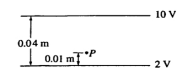Two large, flat, parallel, conducting plates are 0.04 m apart, as shown above. The lower plate is at a
potential of 2 V with respect to ground. The upper plate is at a potential of 10 V with respect to
ground. Point P is located 0.01 m above the lower plate.The electric potential at point P is
(A) 10 V (B) 8 V (C) 6 V (D) 4 V

(D)

25

A particle of charge Q and mass m is accelerated from rest through a potential difference V, attaining a kinetic energy K. What is the kinetic energy of a particle of charge 2Q and mass m/2 that is accelerated from rest through the same potential difference?
(A) ½ K (B) K (C) 2K (D) 4K

(C)

26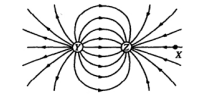The diagram above shows electric field lines in an isolated region of space containing two small charged spheres, Y and Z. Which of the following statements is true?
(A) The charge on Y is negative and the charge on Z is positive.
(B) The strength of the electric field is the same everywhere.
(C) The electric field is strongest midway between Y and Z.
(D) A small negatively charged object placed at point X would tend to move toward the right.

(D)

27

A parallel–plate capacitor has a capacitance Co. A second parallel–plate capacitor has plates with twice the area and twice the separation. The capacitance of the second capacitor is most nearly
(A) ¼Co (B) ½Co (C) Co (D) 2Co

(C)

28

The electric field E just outside the surface of a charged conductor is
(A) directed perpendicular to the surface

(B) directed parallel to the surface

(C) zero

(D) infinite

(A)

29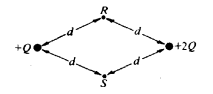Points R and S are each the same distance d from two unequal charges, +Q and +2Q, as shown above. The work required to move a charge –Q from point R to point S is
(A) dependent on the path taken from R to S
(B) positive
(C) zero
(D) negative

(C)

30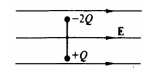A rigid insulated rod, with two unequal charges attached to its ends, is placed in a uniform electric field E as shown above. The rod experiences a
(A) net force to the left and a clockwise rotation
(B) net force to the left and a counterclockwise rotation
(C) net force to the right and a clockwise rotation
(D) net force to the right and a counterclockwise rotation

(B)

31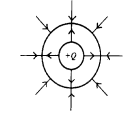The electric field of two long coaxial cylinders is represented by lines of force as shown above. The charge on the inner cylinder is +Q. The charge on the outer cylinder is
(A) +3Q (B) +Q (C) – Q (D) –3 Q

(D)

32

An isolated capacitor with air between its plates has a potential difference Vo and a charge Qo. After the space between the plates is filled with oil, the difference in potential is V and the charge is Q. Which of the following pairs of relationships is correct?
(A) Q = Qo and V > Vo

(B) Q = Qo and V < Vo

(C) Q > Qo and V = Vo

(D) Q < Qo and V < Vo

(B)

33

Two small spheres have equal charges q and are separated by a distance d. The force exerted on each sphere by the other has magnitude F. If the charge on each sphere is doubled and d is halved, the force on each sphere
has magnitude
(A) F (B) 4F (C) 8F (D) 16F

(D)

34

Which of the following statements about conductors under electrostatic conditions is true?
(A) Positive work is required to move a positive charge over the surface of a conductor.
(B) Charge that is placed on the surface of a conductor always spreads evenly over the surface.
(C) The electric potential inside a conductor is always zero.
(D) The surface of a conductor is always an equipotential surface.

(D)

35

A charged particle traveling with a velocity v in an electric field E experiences a force F that must be
(A) parallel to v

(B) perpendicular to v

(C) parallel to E

(D) perpendicular to E

(C)

36

A positive charge of 3.0 × 10–8 coulomb is placed in an upward directed uniform electric field of 4.0 × 104 N/C. When the charge is moved 0.5 meter upward, the work done by the electric force on the charge is
(A) 6 × 10–4 J

(B) 12 × 10–4 J

(C) 2 × 104 J

(D) 8 × 104 J

(A)

37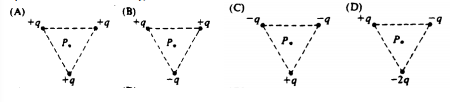The following configurations of electric charges are located at the vertices of an equilateral triangle. Point P is equidistant from the charges.

In which configuration is the electric field at P equal to zero?
(A) A (B) B (C) C (D) D

(A)

38The following configurations of electric charges are located at the vertices of an equilateral triangle. Point P is equidistant from the charges.

In which configuration is the electric field at P pointed at the midpoint between two of the charges?
(A) A (B) B (C) C (D) D

(C)

39

A sheet of mica is inserted between the plates of an isolated charged parallel–plate capacitor. Which of the following statements is true?
(A) The capacitance decreases.
(B) The potential difference across the capacitor decreases.
(C) The charge on the capacitor plates decreases
(D) The electric field between the capacitor plates increases.

(B)

40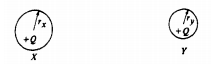Two conducting spheres, X and Y have the same positive charge +Q, but different radii
(rx > ry) as shown above. The spheres are separated so that the distance between them is large compared with either radius. If a wire is connected between them, in which direction will electrons be directed in the wire?
(A) From X to Y
(B) From Y to X
(C) There will be no flow of charge in the wire.
(D) It cannot be determined without knowing the magnitude of Q.

(A)

41

A sphere of radius R has positive charge Q uniformly distributed on its surface.

Which of the following represents the magnitude of the electric field E and the potential V as functions of r, the distance from the center of the sphere, when r < R ?
E V
(A) 0 kQ/R
(B) 0 kQ/r
(C) kQ/r2 0
(D) kQ/R2 0

(A)

42

A sphere of radius R has positive charge Q uniformly distributed on its surface.

Which of the following represents the magnitude, of the electric field E and the potential V as functions of r, the distance from the center of sphere, when r > R ?

E V
(A) kQ/R2 kQ/R
(B) kQ/R kQ/r
(C) kQ/r2 kQ/r
(D) kQ/r2 kQ/r

(C)

43

From the electric field vector at a point, one can determine which of the following?
I. The direction of the electrostatic force on a test charge of known sign at that point
II. The magnitude of the electrostatic force exerted per unit charge on a test charge at that point
III. The electrostatic charge at that point
(A) I only

(B) III only

(C) I and II only

(D) II and III only

(C)

44

A conducting sphere of radius R carries a charge Q. Another conducting sphere has a radius R/2, but carries the same charge. The spheres are far apart. The ratio of the electric field near the surface of the smaller sphere to
the field near the surface of the larger sphere is most nearly
(A) 1/4 (B) 1/2 (C) 2 (D) 4

(D)

45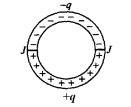A circular ring made of an insulating material is cut in half. One half is given a charge –q uniformly distributed along its arc. The other half is given a charge + q also uniformly distributed along its arc. The two halves are then rejoined with insulation at the junctions J, as shown above. If there is no change in the charge distributions, what is the direction of the net electrostatic force on an electron located at the center of the circle?
(A) Toward the top of the page

(B) Toward the bottom of the page

(C) To the right

D) To the left

(B)

46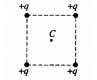Four positive charges of magnitude q are arranged at the corners of a square, as shown above. At the center C of the square, the potential due to one charge alone is Vo and the electric field due to one charge alone has magnitude Eo. Which of the following correctly gives the electric potential and the magnitude of the electric field at the center of the square due to all four charges?
Electric Potential Electric Field
(A) Zero Zero
(B) Zero 2Eo

(C) 4 Vo Zero
(D) 4 Vo 2Eo

(C)

47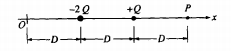Two charges, –2Q and +Q, are located on the x–axis, as shown above. Point P, at a distance of 3D from the origin O, is one of two points on the positive x–axis at which the electric potential is zero. How far from the origin O is the other point?
(A) 2/3 D

(B) 3/2 D

(C) 5/3 D

(D) 2D

(C)

48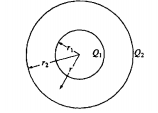Two concentric, spherical conducting shells have radii r1 and r2 and charges Q1 and Q2, as shown above. Let r be the distance from the center of the spheres and consider the region r1 < r < r2. In this region the electric field is proportional to
(A) Q1/r2

(B) (Q1 + Q2)/r2

(C) (Q1 + Q2)/r

(D) Q1/r + Q2/r2

(A)

49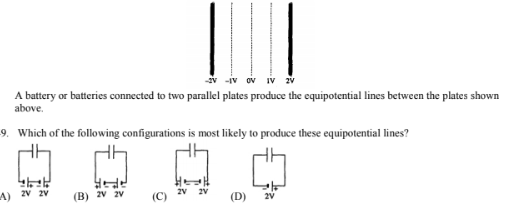Which of the following configurations is most likely to produce these equipotential lines?

(A)
(B)
(C)
(D)

(A)

50

The force on an electron located on the 0 volt potential line is:
(A) 0 N

(B) 1 N, directed to the right

(C) 1 N, directed to the left

(D) to the right, but its magnitude cannot be determined without knowing the distance between the lines

(D)

51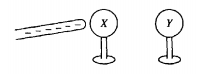Two metal spheres that are initially uncharged are mounted on insulating stands, as shown above. A negatively
charged rubber rod is brought close to, but does not make contact with, sphere X. Sphere Y is then brought close to X on the side opposite to the rubber rod. Y is allowed to touch X and then is removed some distance away. The rubber rod is then moved far away from X and Y. What are the final charges on the spheres?
Sphere X Sphere Y
A) Zero Zero
B) Negative Positive
C) Positive Negative
D) Positive Positive

(C)

52

Two initially uncharged conductors, 1 and 2, are mounted on insulating stands and are in contact, as shown above. A negatively charged rod is brought near but does not touch them. With the rod held in place, conductor 2 is moved to the right by pushing its stand, so that the conductors are separated. Which of the following is now true of conductor 2?
(A) It is uncharged.

(B) It is positively charged.

(C) It is negatively charged.

(D) It is charged, but its sign cannot be predicted.

(C)

53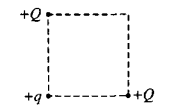As shown above, two particles, each of charge +Q, are fixed at opposite corners of a square that lies in the plane of the page. A positive test charge +q is placed at a third corner. What is the direction of the force on the test charge due to the two other charges?

(A) ↘
(B) ↗
(C) ↓
(D) ↙

(D)

54

If F is the magnitude of the force on the test charge due to only one of the other charges, what is the magnitude of the net force acting on the test charge due to both of these charges?

(A) Zero

(B) F/ √2

(D) √2F

(E) 2

(D)

55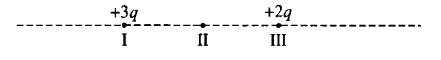Two charges are located on the line shown in the figure below, in which the charge at point I is +3q and the charge at point III is +2q. Point II is halfway between points I and III.

Other than at infinity, the electric field strength is zero at a point on the line in which of the following ranges?

1. To the left of I
2. Between I and II
3. Between II and III
4. To the right of III

(C)

56

Two charges are located on the line shown in the figure below, in which the charge at point I is +3q and the charge at point III is +2q. Point II is halfway between points I and III.

The electric potential is negative at some points on the line in which of the following ranges?
(A) To the left of I

(B) Between I and II

(C) Between II and III

(D) None; this potential is never negative.

(D)

57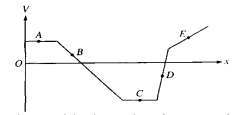The graph above shows the electric potential V in a region of space as a function of position along the x–axis.
At which point would a charged particle experience the force of greatest magnitude?
(A) A (B) B (C) D (D) E

(C)

58

If the only force acting on an electron is due to a uniform electric field, the electron moves with constant
(A) acceleration in a direction opposite to that of the field
(B) acceleration in the direction of the field
(C) speed in a direction opposite to that of the field
(D) speed in the direction of the field

(A)

59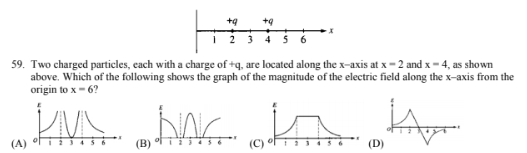Two charged particles, each with a charge of +q, are located along the x–axis at x = 2 and x = 4, as shown above. Which of the following shows the graph of the magnitude of the electric field along the x–axis from the origin to x = 6?

(A)

60

A positive electric charge is moved at a constant speed between two locations in an electric field, with no work done by or against the field at any time during the motion. This situation can occur only if the:
(A) charge is moved in the direction of the field
(B) charge is moved opposite to the direction of the field
(C) charge is moved perpendicular to an equipotential line
(D) charge is moved along an equipotential line

(D)

61

The nonconducting hollow sphere of radius R shown above carries a large charge +Q, which is uniformly
distributed on its surface. There is a small hole in the sphere. A small charge +q is initially located at point P a distance r from the center of the sphere. If k = 1/4πεo, what is the work that must be done by an external agent in moving the charge +q from P through the hole to the center O of the sphere?
(A) kqQ/r

(B) kqQ/R

(C) kq(Q – q)/r

(D) kqQ(1/R – 1/r)

(D)

62

A capacitor is constructed of two identical conducting plates parallel to each other and separated by a distance d. The capacitor is charged to a potential difference of V0 by a battery, which is then disconnected

If any edge effects are negligible, what is the magnitude of the electric field between the plates?
(A) V0d

(B) V0/d

(C) V0/d2

(D) V0 2/d

(B)

63

A capacitor is constructed of two identical conducting plates parallel to each other and separated by a distance d. The capacitor is charged to a potential difference of V0 by a battery, which is then disconnected.

A sheet of insulating plastic material is inserted between the plates without otherwise disturbing the system. What effect does this have on the capacitance?
(A) It causes the capacitance to increase.
(B) It causes the capacitance to decrease.
(C) None; the capacitance does not change.
(E) Nothing can be said about the effect without knowing the thickness of the sheet.

(A)

64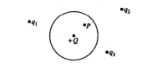A point charge +Q is inside an uncharged conducting spherical shell that in turn is near several isolated point charges, as shown above. The electric field at point P inside the shell depends on the magnitude of:
(A) Q only
(B) the charge distribution on the sphere only
(C) Q and the charge distribution on the sphere
(D) all of the point charges

(A)

65

A potential difference V is maintained between two large, parallel conducting plates. An electron starts from
rest on the surface of one plate and accelerates toward the other. Its speed as it reaches the second plate is
proportional to
(A) 1/ √V

(B) √V

(C) V

(D) V2

(B)

66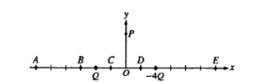Particles of charge Q and –4Q are located on the x–axis as shown in the figure above. Assume the particles are isolated from all other charges.

66. Which of the following describes the direction of the electric field at point P?
(A) +y

(B)–y

(C) Components in both the –x and +y directions

(D) Components in both the +x and –y directions

(D)

67Particles of charge Q and –4Q are located on the x–axis as shown in the figure above. Assume the particles are isolated from all other charges.

At which of the labeled points on the x–axis is the electric field zero?
(A) A (B) B (C) C (D) E

(A)

68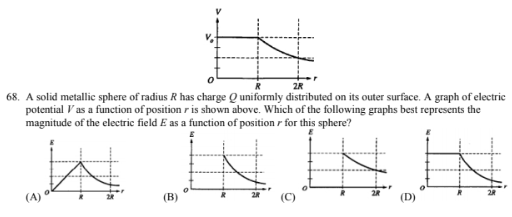A solid metallic sphere of radius R has charge Q uniformly distributed on its outer surface. A graph of electric potential V as a function of position r is shown above. Which of the following graphs best represents the magnitude of the electric field E as a function of position r for this sphere?

(B)

69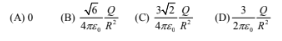As shown in the figure above, six particles, each with charge +Q, are held fixed and ate equally spaced around the circumference of a circle of radius R.

69. What is the magnitude of the resultant electric field at the center of the circle?

(A)

(B)

(C)

(D)

(A)

70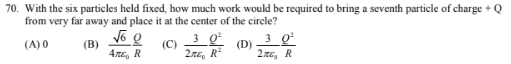As shown in the figure above, six particles, each with charge +Q, are held fixed and ate equally spaced around the circumference of a circle of radius R.

With the six particles held fixed, how much work would be required to bring a seventh particle of charge + Q from very far away and place it at the center of the circle?

(A)
(B)
(C)
(D)

(D)

71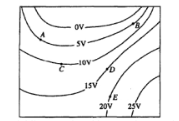The diagram above shows equipotential lines produced by an unknown charge distribution. A, B, C, D, and E
are points in the plane.
71. Which vector below best describes the direction of the electric field at point A ?
(A)↗
(B)↙
(C)↘
(D)↖

(A)

72At which point does the electric field have the greatest magnitude?
(A) A (B) B (C) C (D) E

(B)

73How much net work must be done by an external force to move a –1 μC point charge from rest at point C to rest at point E ?
(A) –20 μJ (B) –10 μJ (C) 10 μJ (D) 20 μJ

(B)

74

A physics problem starts: "A solid sphere has charge distributed uniformly throughout. . . " It may be correctly
concluded that the
(A) electric field is zero everywhere inside the sphere
(B) electric potential on the surface of the sphere is not constant
(C) electric potential in the center of the sphere is zero
(D) sphere is not made of metal

(D)

75

A uniform spherical charge distribution has radius R.. Which of the following is true of the electric field strength due to this charge distribution at a distance r from the center of the charge?
(A) It is greatest when r = 0.
(B) It is directly proportional to r when r > R.
(C) It is directly proportional to r when r < R.
(D) It is directly proportional to r2

(C)

76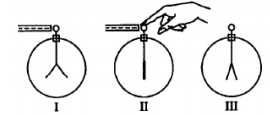When a negatively charged rod is brought near, but does not touch, the initially uncharged electroscope shown above, the leaves spring apart (I). When the electroscope is then touched with a finger, the leaves collapse (II). When next the finger and finally the rod are removed, the leaves spring apart a second time (III). The charge on the leaves is:
(A) positive in both I and III
(B) negative in both I and III
(C) positive in I, negative in III
(D) negative in I, positive in III

(B)

77

A positively charged conductor attracts a second object. Which of the following statements could be true?
I. The second object is a conductor with negative net charge.
II. The second object is a conductor with zero net charge.
III. The second object is an insulator with zero net charge..
(A) I only (B) II only (C) III only (D) I, II & III

(D)

78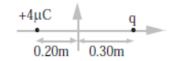A point charge of +4.0 μC is placed on the negative x–axis 0.20 m to the left of the origin, as shown in the accompanying figure. A second point charge q is placed on the positive x–axis 0.30 m to the right of the origin.

If the net electric field at the origin is zero. What is q?
(A) +9.0 μC
(B) +6.0 μC
(C) –6.0 μC
(D) –9.0 μC

(A)

79A point charge of +4.0 μC is placed on the negative x–axis 0.20 m to the left of the origin, as shown in the accompanying figure. A second point charge q is placed on the positive x–axis 0.30 m to the right of the origin.

If the net electric potential at the origin is zero. What is q?
(A) +9.0 μC
(B) +6.0 μC
(C) –6.0 μC
(D) –9.0 μC

(C)

80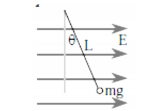A small object with charge q and weight mg is attached to one end of a string of length L. The other end is attached to a stationary support. The system is placed in a uniform horizontal electric field E, as shown in the accompanying figure. In the presence of the field, the string makes a constant angle q with the vertical. What is the sign and magnitude of q?

(A) positive with magnitude mg/E
(B) negative with magnitude mg/E
(C) positive with magnitude (mg/E)tanθ
(D) negative with magnitude (mg/E)tanθ

(C)

81

Two large parallel plates a distance d apart are charged by connecting them to a battery of potential difference V. The battery is disconnected, and the plates are slowly moved apart. As the distance between plates increases:

(A) the charge on the plates decreases.
(B) the electric field intensity between the plates increases.
(C) the potential difference between the plates decreases.
(D) the potential difference between the plates increases.

(D)

82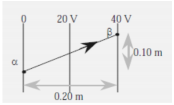In the figure above, equipotential lines are drawn at 0, 20.0 V, and 40.0 V. The total work done in moving a point charge of + 3.00 mC from position a to position b is:
(A) 4.00 mJ
(B) 8.00 mJ
(C) 12.0 mJ
(D) 120 mJ

(D)

83

Two positive point charges repel each other with force 0.36 N when their separation is 1.5 m. What force do they exert on each other when their separation is 1.0 m?
(A) 0.81 N
(B) 0.54 N
(C) 0.24 N
(D) 0.16 N

(A)

84

Two isolated conducting spheres (S1 of radius 0.030 m and initial charge + 6.0 nC and S2 of radius 0.040 m and initial charge + 2.0 nC) are connected by a conducting wire. Charge will flow in the wire until:
(A) both spheres are equally charged.
(B) the force of repulsion between the two spheres becomes equal.
(C) both spheres have the same surface charge density.
(D) both spheres are at the same potential.

(D)

85

A point charge +q is placed midway between two point charges +3q and –q separated by a distance 2d. If Coulomb’s constant is k, the magnitude of the force on the charge +q is:

(A) 2kq2/d2
(B) 4kq2/d2
(C) 6kq2/d2
(D) 8kq2/d2

(B)

86A charged rod is placed between two insulated conducting spheres as shown. The spheres have no net charge.

Region II has the same polarity as Region:

(A) I only (B) III only (C) IV only (D) I & IV only

(B)

87

Two large oppositely charged insulated plates have a uniform electric field between them. The distance between the plates is increased. Which of the following statements is true?

I. The field strength decreases.
II. The field strength increases.
III. The potential difference between the plates increases.

(A) I only (B) II only (C) III only (D) I and III only

(C)

88

When two charged point–like objects are separated by a distance R, the force between them is F. If the distance between them is quadrupled, the force between them is:

(A) 16 F (B) 4 F (C) F/4 (D) F/16

(D)

89

An electroscope is given a positive charge, causing its foil leaves to separate. When an object is brought near the top plate of the electroscope, the foils separate even further. We could conclude:

(A) that the object is positively charged.
(B) that the object is negatively charged.
(C) only that the object is charged.
(D) only that the object is uncharged.

(A)

90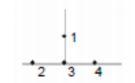Four positive point charges are arranged as shown in the accompanying diagram. The force between charges 1 and 3 is 6.0 N; the force between charges 2 and 3 is 5.0 N; and the force between charges 3 and 4 is 3.0 N. The magnitude of the total force on charge 3 is most nearly:

(A) 6.3 N (B) 8.0 N (C) 11 N (D) 14 N

(A)

91

Two isolated parallel plates are separated by a distance d. They carry opposite charges Q and each has surface area A. Which of the following would increase the strength of the electric field between the plates?

I. Increasing Q
II. Increasing A
III. Increasing d

(A) I only (B) II only (C) III only (D) II & III only

(A)

92

When a positive electrically charged glass rod is brought near a neutral hollow metal sphere suspended by an insulating string, the sphere will be attracted to the rod because:

(A) the rod removes electron from the sphere
(B) the electric charge produces a magnetic field to attract the sphere
(C) the charge on the rod causes a separation of charge in the sphere
(D) some of the protons from the rod have been given to the sphere

(C)

93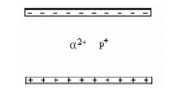An alpha particle and a proton are placed equal distance between two large charged metal plates as shown. Which of the following would best describe the motion of the two particles if they were free to move?

(A) The alpha particle will travel upwards with twice the velocity of the proton.
(B) Both particles will travel upwards with the same velocity.
(C) Both particles will accelerate upwards with the same acceleration.
(D) The alpha particle will accelerate upwards with half the acceleration of the proton

(D)

94

Two parallel metal plates carry opposite electrical charges each with a magnitude of Q. The plates are separated by a distance d and each plate has an area A. Consider the following:
I. increasing Q
II. increasing d
III. increasing A

Which of the following would have the effect of reducing the potential difference between the plates?
(A) I only (B) II only (C) III only (D) II and III

(C)

95

A positive point charge of +q and a negative point charge of –q are separated by a distance d. What would be the magnitude of the electric field midway between the two charges?

(A) E = kq/d2
(B) E = 2kq/d2
(C) E = 4kq/d
(D) E = 8kq/d2

(D)

96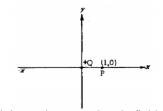A positive charge +Q located at the origin produces an electric field E0 at point P (x = +1, y = 0). A negative charge –2Q is placed at such a point as to produce a net field of zero at point P. The second charge will be placed on the:

(A) x-axis where x > 1
(B) x-axis where x < 0
(C) y-axis where y > 0
(D) y-axis where y < 0

(B)

97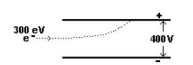A 300 eV electron is aimed midway between two parallel metal plates with a potential difference of 400 V. The electron is deflected upwards and strikes the upper plate as shown. What would be the kinetic energy of the electron just before striking the metal plate?

(A) 360 eV
(B) 400 eV
(C) 500 eV
(D) 700 eV

(C)

98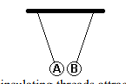Two small hollow metal spheres hung on insulating threads attract one another as shown. It is known that a positively charged rod will attract ball A.
I. Ball A has a positive charge
II. Ball B has a negative charge
III. Ball A and Ball B have opposite charges

Which of the above can be correctly concluded about the charge on the balls?

(A) I only (B) II only (C) III only (D) none of these

(D)

99

A 5x10-6 coulomb electric charge is placed midway between two parallel metal plates connected to a 9–volt battery. If the electric charge experiences a force of 1.5x10–4 newtons, what is the separation of the metal plates?

(A) 6.75 × 10-9 m
(B) 2.7 × 10-4 m
(C) 3.7 × 10-3 m
(D) 0.30 m

(D)

100

A parallel–plate capacitor is connected with wires of negligible resistance to a battery having emf E until the capacitor is fully charged. The battery is then disconnected from the circuit and the plates of the capacitor are moved to half of their original separation using insulated gloves. Let Vnew be the potential difference across the
capacitor plates when the plates are moved together. Let Vold be the potential difference across the capacitor plates when connected to the battery.
Vnew / Vold =
(A) ½
(B) 1
(C) 2
(D) 4

(A)

101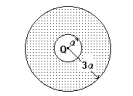A solid, uncharged conducting sphere of radius 3a contains a hollowed spherical region of radius a. A point charge +Q is placed at the common center of the spheres. Taking V = 0 as r approaches infinity, the potential at position r = 2 a from the center of the spheres is:
(A) 0
(B) 2kQ/3a
(C) kQ/3a
(D) kQ/a

(C)

102

Two identical electrical point charges Q, separated by a distance d produce an electrical force of F on one another. If the distance is decreased to a distance of 0.40d, what is the strength of the resulting force?
(A) 6.3F
(B) 2.5F
(C) 0.40F
(D) 0.16F

(A)

103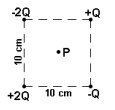Four electrical charges are arranged on the corners of a 10 cm square as shown.
What would be the direction of the resulting electric field at the center point P?

(A) →
(B) ↑
(C) ↓
(D) ↗

(B)

104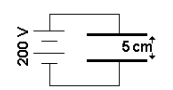A proton is released between the two parallel plates of the fully charged capacitor shown above. What would be the resulting acceleration of the proton?
(A) 7.3 × 1013 m/s2
(B) 9.6 × 108 m/s2
(C) 6.3 × 1019 m/s2
(D) 3.8 × 1011 m/s2

(D)

105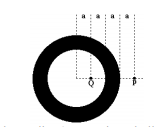A solid uncharged conducting sphere has radius 3a contains a hollowed spherical region of radius 2a. A point charge +Q is placed at a position a distance a from the common center of the spheres. What is the magnitude of the electric field at the position r = 4a from the center of the spheres as marked in the figure by P?

(A) 0
(B) kQ/16a2
(C) 3kQ/16a2
(D) kQ/9a2

(B)

106

A positively charged object is brought near but not in contact with the top of an uncharged gold leaf electroscope. The experimenter then briefly touches the electroscope with a finger. The finger is removed, followed by the removal of the positively charged object. What happens to the leaves of the electroscope when a negative charge is now brought near but not in contact with the top of the electroscope?

(A) they remain uncharged
(B) they move farther apart
(C) they move closer together
(D) they remain negatively charged but unmoved

(B)

107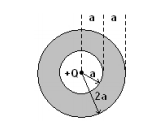A solid spherical conducting shell has inner radius a and outer radius 2a. At the center of the shell is located a point charge +Q. What must the excess charge of the shell be in order for the charge density on the inner and outer surfaces of the shell to be exactly equal?
(A) –5Q
(B) +3Q
(C) –4Q
(D) +4Q

(A)

108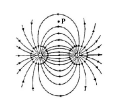A small positive test charge is placed at point P in the region near two charges. Which of the following arrows indicates the direction of the force on the positive test charge?

(A) →
(B) ↓
(C) ←
(D) ↘

(C)

109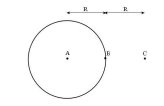A spherical conducting shell has a net charge +Q placed on it. Which of the following is the correct
relationship for the electric potential at the points labeled A, B, and C? Point A is at the center of the sphere, point B is at the surface of the shell, a distance R from point A, and point C is a distance R from point B outside the sphere. As r goes to infinity, V = 0.

(A) VC < VB < VA
(B) VA < VB < VC
(C) VC = VB = VA
(D) VC < VB = VA

(D)

110

Which statement about a system of point charges that are fixed in space is necessarily true?

(A) If the potential energy of the system is negative, net positive work by an external agent is required to take the charges in the system back to infinity.

(B) If the potential energy of the system is positive, net positive work is required to bring any new charge not part of the system in from infinity to its final resting location.

(C) If the potential energy of the system is zero, no negative charges are in the configuration.

(D) If the potential energy of the system is negative, net positive work by an external agent was required to assemble the system of charges.

(A)

111

A positive point charge exerts a force of magnitude F on a negative point charge placed a distance x away. The negative point charge is replaced with a positive point charge and the distance between the two point charges is
halved. What is the magnitude of the new force that the positive point charge exerts on the negative point charge?

(A) 4F
(B) 2F
(C) F
(D) F/2

(A)

112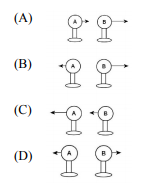Two uniformly charged non–conducting spheres on insulating bases are placed on an air table. Sphere A has a charge +3Q coulombs and sphere B has a charge +Q coulombs. Which of the following correctly illustrates the magnitude and direction of the electrostatic force between the spheres when they are released?

(A)
(B)
(C)
(D)

(D)

113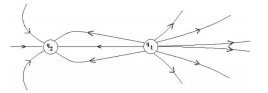For the diagram shown above, what is the ratio of the charges q2/q1 where the diagram shown has a representation of the field lines in the space near the charges?

(A) -3/2
(B) -2/3
(C) 2/3
(D) 3/2

(B)

114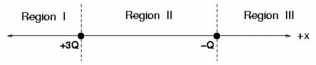Two point charges are fixed on the x–axis in otherwise empty space as shown above.

In which Region(s) is there a place on the x–axis (aside from infinity) at which the electric potential is equal to zero?

(A) Only in Region II
(B) In both Regions I and II
(C) In both Regions I and III
(D) In both Regions II and III

(D)

115Two point charges are fixed on the x–axis in otherwise empty space as shown above.

In which Region(s) is there a place on the x–axis (aside from infinity) at which the electric field is equal to zero?

(A) Only in Region I
(B) In both Regions I and II
(C) Only in Region III
(D) In both Regions II and III

(C)

116

A parallel–plate capacitor is connected to a battery. Without disconnecting the capacitor, a student pulls the capacitor’s plates apart so that the plate separation doubles. As a result of this action, what happens to the voltage across the capacitor and the energy stored by the capacitor?

(A) the voltage doubles; the energy stays the same
(B) the voltage doubles; the energy halves
(C) the voltage stays the same; the energy halves
(D) the voltage stays the same; the energy doubles

(C)

117

A person rubs a neutral comb through their hair and the comb becomes negatively charged. Which of the following is the best explanation for this phenomenon?

(A) The hair gains protons from the comb.
(B) The hair gains protons from the comb while giving electrons to the comb.
(C) The hair loses electrons to the comb.
(D) The comb loses protons to the person’s hand holding the comb.

(C)

118A charge of +Q is located on the x–axis at x = –1 meter and a charge of –2Q is held at x = +1 meter, as shown in the diagram above. At what position on the x–axis will a test charge of +q experience a zero net electrostatic force?

(A) – 3 √8 m (B) –1/3 m (C) 1/3 m (D) 3 √8 m

(A)

119

The two plates of a parallel-plate capacitor are a distance d apart and are mounted on insulating supports. A battery is connected across the capacitor to charge it and is then disconnected. The distance between the insulated plates is then increased to 2d. If fringing of the field is still negligible, which of the following quantities is doubled?

(A) The capacitance of the capacitor
(B) The surface density of the charge on the plates of the capacitor
(C) The energy stored in the capacitor
(D) The intensity of the electric field between the plates of the capacitor

(C)

120

Two point objects each carrying charge 10Q are separated by a distance d. The force between them is F. If half the charge on one object is transferred to the other object while at the same time the distance between them is
doubled, what is the new force between the two objects?

(A) 0.19 F (B) 0.25 F (C) 0.75 F (D) 4.0 F

(A)

121

Two identical spheres carry identical electric charges. If the spheres are set a distance d apart they repel one another with a force F. A third sphere, identical to the other two but initially uncharged is then touched to one sphere and then to the other before being removed. What would be the resulting force between the original two spheres?
(A) ¾ F (B) 5/8 F (C) ½ F (D) 3/8 F

(D)

122

An alpha particle is accelerated to a velocity v in a particle accelerator by a potential difference of 1200 V.

Which of the following potential differences would be needed to give the alpha particle twice the velocity?

(A) 4800 V (B) 4100 V (C) 2400 V (D) 1700 V

(A)

123

An electrical charge Q is placed at one vertex of an equilateral triangle. When an identical charge is placed at another vertex, each charge feels a force of 15 N. When a third charge identical to the first two, is placed at the third vertex, what would be the magnitude of the force on each charge?

(A) 15 N (B) 26 N (C) 30 N (D) 42 N

(B)

124

Two conducting spheres with the same charge Q are separated by an infinite distance. Sphere A has a radius of 10 cm while sphere B has a radius of 20 cm. At what distance from the centers of the spheres would the magnitude of the electric field be the same?

(A) 15 cm from A and 15 cm from B
(B) 30 cm from A and 40 cm from B
(C) 20 cm from A and 40 cm from B
(D) 40 cm from A and 40 cm from B

(D)

125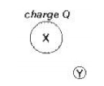A large conducting sphere labeled X contains an electrical charge Q. Sphere X is connected by a metal wire to a small uncharged conducting sphere labeled Y. The wire is then removed. How does the electrical field (Ey) at the surface of sphere Y compare to the electrical field (Ex) at the surface of sphere X?
(A) Ex = Ey = 0
(B) Ey= Ex ≠0
(C) Ey< Ex
(D) Ey> Ex

(D)

126

What voltage would be required across a 8.9 nF capacitor to accumulate 1.5 × 1012 excess electrons on one plate of the capacitor?

(A) 3.7 V (B) 5.9 V (C) 14 V (D) 27 V

(D)

127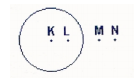A hollow metal sphere is uniformly charged with positive charge. Points K and L are inside the sphere and points M and N are outside the sphere as shown in the diagram. At which pair of points would the field be the smallest?
(A) points K and N
(B) points L and M
(C) points K and L
(D) points M and N

(C)

128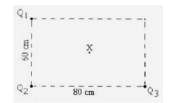Three electric charges (Q1, Q2, and Q3) are arranged at three corners of a rectangle as shown in the diagram and each has a charge of -40 nC.

What is the magnitude of the net force on Q2?

(A) 1.4 × 10-5 N
(B) 1.7 × 10-5 N
(C) 4.2 × 10-5 N
(D) 4.6 × 10-5 N

(D)

129Three electric charges (Q1, Q2, and Q3) are arranged at three corners of a rectangle as shown in the diagram and each has a charge of -40 nC.

What would be the magnitude of the total electric field at center point X?

(A) 1440 N/C
(B) 720 N/C
(C) 360 N/C
(D) 180 N/C

(A)

130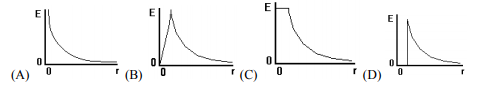Which of the following graphs would best represent the electric field of a hollow sphere as a function of distance from its center when it is charged to a potential of 400,000 volts?

(A)
(B)
(C)
(D)

(D)

131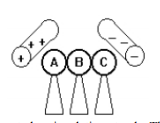Three metal spheres A, B, and C are mounted on insulating stands. The spheres are touching one another, as shown in the diagram below. A strong positively charged object is brought near sphere A and a strong negative charge is brought near sphere C. While the charged objects remain near spheres A and C, sphere B is removed by means of its insulating stand. After the charged objects are removed, sphere B is first touched to sphere A and then to sphere C. The resulting charge on B would be of what relative amount and sign?

(A) the same sign but 1/2 the magnitude as originally on sphere A
(B) the opposite sign but 1/2 the magnitude as originally on sphere A
(C) the opposite sign but 1/4 the magnitude as originally on sphere A
(D) the same sign but 1/2 the magnitude as originally on sphere C

(C)

132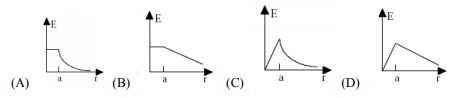A charge is uniformly distributed through a volume of radius a. Which of the graphs below best represents the magnitude of the electric field as a function of distance from the center of the sphere?

(A)
(B)
(C)
(D)

(C)

133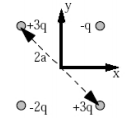Four point charges are placed at the corners of a square with diagonal 2a as shown in the diagram. What is the total electric field at the center of the square?

(A) kq/a2 at an angle 45° above the +x axis.
(B) kq/a2 at an angle 45° below the –x axis.
(C) 3kq/a2 at an angle 45° above the –x axis.
(D) 3kq/a2 at an angle 45° below the +x axis.

(B)

134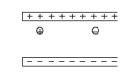A free electron and a free proton are placed between two oppositely charged parallel plates. Both are closer to the positive plate than the negative plate. See the diagram below. Which of the following statements is true?

I. The force on the proton is greater than the force on the electron.
II. The potential energy of the proton is greater than that of the electron.
III. The potential energy of the proton and the electron is the same.

(A) II only (B) III only (C) I & II only (D) I & III only

(A)

135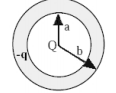A spherical shell with an inner surface of radius a and an outer surface of radius b is made of conducting material. A charge +Q is placed at the center of the spherical shell and a total charge –q is placed on the shell.

How is the charge –q distributed after it has reached equilibrium?

(A) +Q on the inner surface, – q – Q on the outer surface.
(B) The charge –q is spread uniformly between the inner and outer surface.
(C) –Q on the inner surface, – q + Q on the outer surface.
(D) –Q on the inner surface, –q on the outer surface.

(C)

136A spherical shell with an inner surface of radius a and an outer surface of radius b is made of conducting material. A charge +Q is placed at the center of the spherical shell and a total charge –q is placed on the shell.

What is the electrostatic potential at a distance R from the center of the shell, where b < R < a?
(A) kQ/a
(B) kQ/R
(C) k(Q – q)/R
(D) k(Q – q)/b

(D)

137

Conducting sphere X is initially uncharged. Conducting sphere Y has twice the diameter of sphere X and initially has charge q. If the spheres are connected by a long thin wire, which of the following is true once equilibrium has been reached?

(A) Sphere Y has half the potential of sphere X. (B) Spheres X and Y have the same potential.
(C) Sphere Y has half the charge of sphere X.
(D) Spheres X and Y have the same charge

(B)

138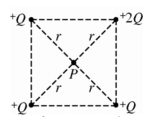Four positive charges are fixed at the corners of a square, as shown above. Three of the charges have magnitude Q, and the fourth charge has a magnitude 2Q. Point P is at the center of the square at a distance r from each charge.

What is the electric potential at point P?
(A) Zero
(B) 2kQ/r
(C) 4kQ/r
(D) 5kQ/r

(D)

139Four positive charges are fixed at the corners of a square, as shown above. Three of the charges have magnitude Q, and the fourth charge has a magnitude 2Q. Point P is at the center of the square at a distance r from each charge.

What is the magnitude of the electric field at point P ?
(A) kQ/r2
(B) 2kQ/r2
(C) 4kQ/r2
(D) 5kQ/r2

(A)

140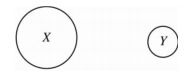The two charged metal spheres X and Y shown above are far apart, and each is isolated from all other charges. The radius of sphere X is greater than that of sphere Y, and the magnitudes of the electric fields just outside their surfaces are the same. How does the charge on sphere X compare with that on sphere Y?

(A) It is greater.
(B) It is less.
(C) It is the same.
(D It cannot be determined without knowing the actual value of the electric field just outside the spheres

(A)

141

Two negative point charges are a distance x apart and have potential energy U. If the distance between the point charges increases to 3x, what is their new potential energy?

(A) 9U (B) 3U (C) 1/3 U (D) 1/9 U

(C)

142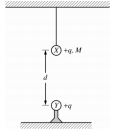Sphere X of mass M and charge +q hangs from a string as shown above. Sphere Y has an equal charge +q and is fixed in place a distance d directly below sphere X. If sphere X is in equilibrium, the tension in the string is most
nearly:

(A) Mg + kq/d
(B) Mg – kq/d
(C) Mg + kq2/d2
(D) Mg – kq2/d2

(D)

143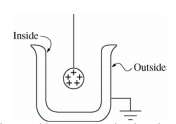A small positively charged sphere is lowered by a nonconducting thread into a grounded metal cup without touching the inside surface of the cup, as shown above. The grounding wire attached to the outside surface is disconnected and the charged sphere is then removed from the cup. Which of the following best describes the subsequent distribution of excess charge on the surface of the cup?

(A) Negative charge resides on the inside surface, and no charge resides on the outside surface.
(B) Negative charge resides on the outside surface, and no charge resides on the inside surface.
(C) Positive charge resides on the inside surface, and no charge resides on the outside surface.
(D) Positive charge resides on the outside surface, and no charge resides on the inside surface

(B)

144

A helium nucleus (charge +2q and mass 4m) and a lithium nucleus (charge +3q and mass 7m) are accelerated through the same electric potential difference, V0. What is the ratio of their resultant kinetic energies, Klithium/Khelium ?

(A) 2/3 (B) 6/7 (C) 7/6 (D) 3/2

(D)

145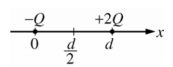A point charge −Q is located at the origin, while a second point charge +2Q is located at x = d on the x-axis, as shown above. A point on the x-axis where the net electric field is zero is located in which of the following regions?

(A) -∞ < x < 0
(B) 0 < x < d/2
(C) d/2 < x < d
(D) d < x < ∞

(A)

146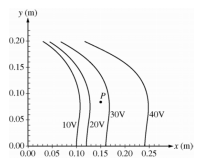A fixed charge distribution produces the equipotential lines shown in the figure above.

Which of the following expressions best represents the magnitude of the electric field at point P ?

(A) 10 V/0.14 m
(B) 10 V/0.04 m
(C) 25 V/0.14 m
(D) 25 V/0.04 m

(B)

147A fixed charge distribution produces the equipotential lines shown in the figure above.

The direction of the electric field at point P is most nearly:

(A) toward the left
(B) toward the right
(C) toward the bottom of the page
(D) toward the top of the page

(A)

148

A cloud contains spherical drops of water of radius R and charge Q. Assume the drops are far apart. If two droplets happen to combine into a single larger droplet, the new potential V at the surface of the larger droplet is most nearly equal to:

(A) 2V0
(B) (2/∛2) V0
(C) ∛2V0
(D) V0

(B)

149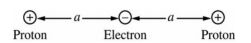Two protons and an electron are assembled along a line, as shown above. The distance between the electron and
each proton is a. What is the work done by an external force in assembling this configuration of charges?

(A) –2ke2/a
(B) –3ke2/2a
(C) ke2/2a
(D) 3ke2/a

(B)